Test: Character Puzzles- 2

# Test: Character Puzzles- 2

Test Description

## 25 Questions MCQ Test | Test: Character Puzzles- 2

Test: Character Puzzles- 2 for Banking Exams 2023 is part of Banking Exams preparation. The Test: Character Puzzles- 2 questions and answers have been prepared according to the Banking Exams exam syllabus.The Test: Character Puzzles- 2 MCQs are made for Banking Exams 2023 Exam. Find important definitions, questions, notes, meanings, examples, exercises, MCQs and online tests for Test: Character Puzzles- 2 below.
Solutions of Test: Character Puzzles- 2 questions in English are available as part of our course for Banking Exams & Test: Character Puzzles- 2 solutions in Hindi for Banking Exams course. Download more important topics, notes, lectures and mock test series for Banking Exams Exam by signing up for free. Attempt Test: Character Puzzles- 2 | 25 questions in 20 minutes | Mock test for Banking Exams preparation | Free important questions MCQ to study for Banking Exams Exam | Download free PDF with solutions
 1 Crore+ students have signed up on EduRev. Have you?
Test: Character Puzzles- 2 - Question 1

### Which one will replace the question mark ?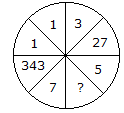Detailed Solution for Test: Character Puzzles- 2 - Question 1

All numbers are cubed,

(7)3 = 343

(1)3 = 1

(3)3 = 27

Similarly, (5)3 = 125.

Test: Character Puzzles- 2 - Question 2

### Which one will replace the question mark ?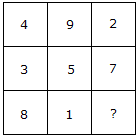Detailed Solution for Test: Character Puzzles- 2 - Question 2

(4 + 9 + 2) = (3 + 5 + 7) = (8 + 1 + 6)

Total in each case = 15.

Test: Character Puzzles- 2 - Question 3

### Which one will replace the question mark ?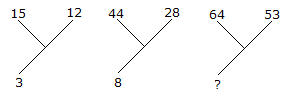Detailed Solution for Test: Character Puzzles- 2 - Question 3

(15 + 12)/9 = 3

and (44 + 28)/9 = 8

Therefore, (64 + 53)/9 = 13.

Test: Character Puzzles- 2 - Question 4

Which one will replace the question mark ?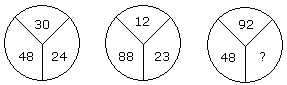Detailed Solution for Test: Character Puzzles- 2 - Question 4

(30 - 24) x 8 = 48

and (23 - 12) x 8 = 88

Therefore, (92 - 86) x 8 = 48.

Test: Character Puzzles- 2 - Question 5

Which one will replace the question mark ?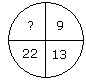Detailed Solution for Test: Character Puzzles- 2 - Question 5

9 + (2)2 = 13

13 + (3)2 = 22

22 + (4)2 = 38.

Test: Character Puzzles- 2 - Question 6

Which one will replace the question mark ?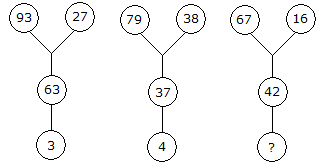Detailed Solution for Test: Character Puzzles- 2 - Question 6

93 - (27 + 3) = 63

79 - (38 + 4) = 37

Therefore, 67 - (16 + X) = 42

X = 9

Test: Character Puzzles- 2 - Question 7

Which one will replace the question mark ?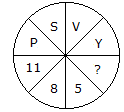Detailed Solution for Test: Character Puzzles- 2 - Question 7

Putting the position of the letters in reverse order

P = 11, S = 8, V = 5 and Y = 2.

Test: Character Puzzles- 2 - Question 8

Which one will replace the question mark ?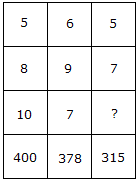Detailed Solution for Test: Character Puzzles- 2 - Question 8

5 x 8 x 10 = 400

and 6 x 9 x 7 = 378

Therefore 5 x 7 x ? = 315

? = 9.

Test: Character Puzzles- 2 - Question 9

Which one will replace the question mark ?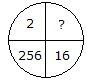Detailed Solution for Test: Character Puzzles- 2 - Question 9

(16)2 = 256

Therefore, (2)2 = 4.

Test: Character Puzzles- 2 - Question 10

Which one will replace the question mark ?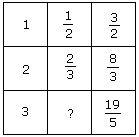Detailed Solution for Test: Character Puzzles- 2 - Question 10

From I row, 1 + (1/2) = 3/2

From II row, 2 + (2/3) = 8/3

From III row, 3 + ? = 19/5

? = (19/5) - 3

? = (4/5).

Test: Character Puzzles- 2 - Question 11

Which one will replace the question mark ?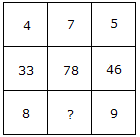Detailed Solution for Test: Character Puzzles- 2 - Question 11

(4 x 8) + 1 = 33

(5 x 9) + 1 = 46

Similarly, (7 x 11) + 1 = 78.

Test: Character Puzzles- 2 - Question 12

Which one will replace the question mark ?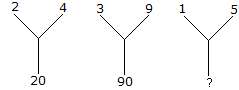Detailed Solution for Test: Character Puzzles- 2 - Question 12

(2)2 + (4)2 = 20

(3)2 + (9)2 = 90

Therefore, (1)2 + (5)2 = 26.

Test: Character Puzzles- 2 - Question 13

Which one will replace the question mark ?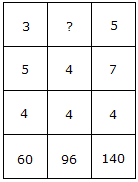Detailed Solution for Test: Character Puzzles- 2 - Question 13

3 x 5 x 4 = 60

and 5 x 7 x 4 = 140

Therefore, 4 x 4 x ? = 96

? = (96/16) = 6.

Test: Character Puzzles- 2 - Question 14

Which one will replace the question mark ?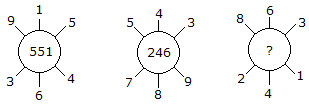Detailed Solution for Test: Character Puzzles- 2 - Question 14

(915 - 364) = 551

(789 - 543) = 246

(863 - 241) = 622.

Test: Character Puzzles- 2 - Question 15

Which one will replace the question mark ?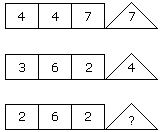Detailed Solution for Test: Character Puzzles- 2 - Question 15

(4 x 7) % 4 = 7

and (6 x 2) % 3 = 4

Therefore, (6 x 2) % 2 = 6.

Test: Character Puzzles- 2 - Question 16

Which one will replace the question mark ?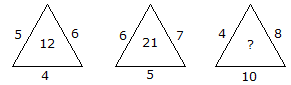Detailed Solution for Test: Character Puzzles- 2 - Question 16

(5 x 6 x 4)/10 = 12

and (6 x 7 x 5)/10 = 21

Therefore (4 x 8 x 10)/10 = 32.

Test: Character Puzzles- 2 - Question 17

Which one will replace the question mark ?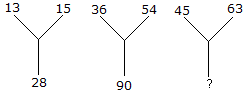Detailed Solution for Test: Character Puzzles- 2 - Question 17

13 + 15 = 28

36 + 54 = 90

Therefore, 45 + 63 = 108.

Test: Character Puzzles- 2 - Question 18

Which one will replace the question mark ?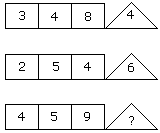Detailed Solution for Test: Character Puzzles- 2 - Question 18

(3 x 4 - 8) = 4

(2 x 5 - 4) = 6

(4 x 5 - 9) = 11.

Test: Character Puzzles- 2 - Question 19

Which one will replace the question mark ?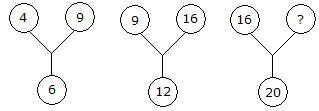Detailed Solution for Test: Character Puzzles- 2 - Question 19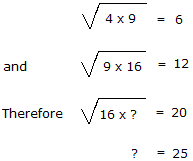Test: Character Puzzles- 2 - Question 20

Which one will replace the question mark ?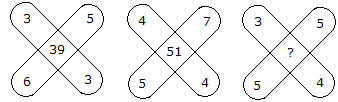Detailed Solution for Test: Character Puzzles- 2 - Question 20

(3 x 3) + (5 x 6) = 39

and (4 x 4) + (5 x 7) = 51

Therefore, (3 x 4) + (5 x 5) = 37.

Test: Character Puzzles- 2 - Question 21

Which one will replace the question mark ?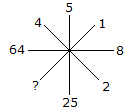Detailed Solution for Test: Character Puzzles- 2 - Question 21

(2)2 = 4

(8)2 = 64

(5)2 = 25

(1)2 = 1.

Test: Character Puzzles- 2 - Question 22

Which one will replace the question mark ?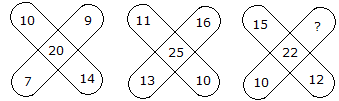Detailed Solution for Test: Character Puzzles- 2 - Question 22

(10 + 9 + 14 + 7)/2 = 20

and (11 + 16 + 10 + 13)/2 = 25

Therefore, (15 + ? + 12 + 10)/2 = 22

Hence 37 + ? = 44

? = 44 - 37

? = 7.

Test: Character Puzzles- 2 - Question 23

Which one will replace the question mark ?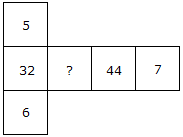Detailed Solution for Test: Character Puzzles- 2 - Question 23

(5 x 6) + 2 = 32

(7 x 6) + 2 = 44

(7 x 5) + 2 = 37.

Test: Character Puzzles- 2 - Question 24

Which one will replace the question mark ?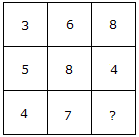Detailed Solution for Test: Character Puzzles- 2 - Question 24

(5 + 3)/2 = 4

and (6 + 8)/2 = 7

Therefore (8 + 4)/2 = 6.

Test: Character Puzzles- 2 - Question 25

Which one will replace the question mark ?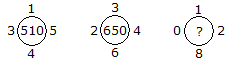Detailed Solution for Test: Character Puzzles- 2 - Question 25

(1)2 + (5)2 + (4)2 + (3)2 = 51 x 10 = 510

and (3)2 + (4)2 + (6)2 + (2)2 = 65 x 10 = 650

Similarly (0)2 + (1)2 + (2)2 + (8)2 = 69 x 10 = 690.

 Use Code STAYHOME200 and get INR 200 additional OFF Use Coupon Code
Information about Test: Character Puzzles- 2 Page
In this test you can find the Exam questions for Test: Character Puzzles- 2 solved & explained in the simplest way possible. Besides giving Questions and answers for Test: Character Puzzles- 2, EduRev gives you an ample number of Online tests for practice